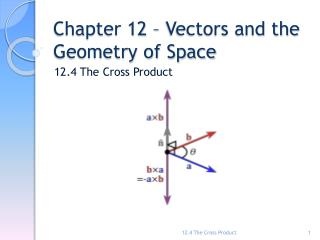DownloadDownload PresentationChapter 12 – Vectors and the Geometry of Space

# Chapter 12 – Vectors and the Geometry of Space

Download Presentation## Chapter 12 – Vectors and the Geometry of Space

- - - - - - - - - - - - - - - - - - - - - - - - - - - E N D - - - - - - - - - - - - - - - - - - - - - - - - - - -
##### Presentation Transcript

1. Chapter 12 – Vectors and the Geometry of Space 12.4 The Cross Product 12.4 The Cross Product

2. Definition – Cross Product Note: The result is a vector. Sometimes the cross product is called a vector product. This only works for three dimensional vectors. 12.4 The Cross Product

3. Cross Product as Determinants • To make Definition one easier, we will use the notation of determinants. A determinant of order 2 is defined by 12.4 The Cross Product

4. Cross Product as Determinants • A determinant of order 3 is defined in terms of second order determinates as shown below. 12.4 The Cross Product

5. Cross Product as Determinants 12.4 The Cross Product

6. Example 1 – pg. 814 #5 • Find the cross product a x b and verify that it is orthogonal to both a and b. 12.4 The Cross Product

7. Theorem 5 • The direction of axb is given by the right hand rule: If your fingers of your right hand curl in the direction of a rotation of an angle less than 180o from a to b, then your thumb points in the direction of axb. 12.4 The Cross Product

8. Visualization • The Cross Product 12.4 The Cross Product

9. Theorems 12.4 The Cross Product

10. Example 2 • For the below problem, find the following: • a nonzero vector orthogonal to the plane through the points P, Q, and R. • the area of triangle PQR. P(2,1,5) Q(-1,3,4) R(3,0,6) 12.4 The Cross Product

11. Theorem 8 • Note: The cross product is not commutative i x j j x i • Associative law for multiplication does not hold. (a x b) x c  a x (b x c) 12.4 The Cross Product

12. Definition – Triple Products • The product a (b x c) is called the scalar triple product of vectors a, b, and c. We can write the scalar triple product as a determinant: 12.4 The Cross Product

13. Definition – Volume of a Parallelepiped 12.4 The Cross Product

14. Example 3 – pg. 815 # 36 • Find the volume of the parallelepiped with adjacent edges PQ, PR, and PS. P(3,0,1) Q(-1,2,5) R(5,1,-1) S(0,4,2) 12.4 The Cross Product

15. Torque • Cross product occurs often in physics. • Let’s consider a force, F, acting on a rigid body at a point given by a position vector r. (i.e. tightening a bolt by applying force to a wrench). The torque  is defined as  = r x F and measures the tendency of the body to rotate about the origin. • The magnitude of the torque vector is ||= |r x F| = |r||F|sin 12.4 The Cross Product

16. Example 4 – pg. 815 #41 • A wrench 30 cm long lies along the positive y-axis and grips a bolt at the origin. A force is applied in the direction <0,3,-4> at the end of the wrench. Find the magnitude of the force needed to supply 100 Nm of torque to the bolt. 12.4 The Cross Product

17. More Examples The video examples below are from section 12.4 in your textbook. Please watch them on your own time for extra instruction. Each video is about 2 minutes in length. • Example 1 • Example 2 • Example 5 12.4 The Cross Product

18. Demonstrations Feel free to explore these demonstrations below. • Cross Product of Vectors 12.4 The Cross Product